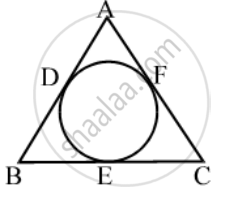# In Fig., if AB = AC, prove that BE = EC - Mathematics

Sum

In Fig., if AB = AC, prove that BE = EC#### Solution

Since tangents from an exterior point to a circle are equal in length.

∴ AD = AF [Tangents from A]

BD = BE [Tangents from B]

CE = CF [Tangents from C]

Now,

AB = AC

⇒ AB – AD = AC – AF [Using (i)]

⇒ BD = CF ⇒ BE = CF [Using (ii)]

⇒ BE = CE [Using (iii)]

Concept: Concept of Circle - Centre, Radius, Diameter, Arc, Sector, Chord, Segment, Semicircle, Circumference, Interior and Exterior, Concentric Circles
Is there an error in this question or solution?